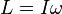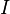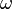# Angular momentum facts for kids

Kids Encyclopedia Facts
(Redirected from Rotational energy)

The angular momentum or rotational momentum (L) of an object rotating about an axis is the product of its moment of inertia and its angular velocity:$L = I \omega$

where$I$ is the moment of inertia (resistance to angular acceleration or deceleration, equal to the product of the mass and the square of its perpendicular distance from the axis of rotation);$\omega \$ is the angular velocity.

There are two kinds of angular momentum: the spin angular momentum and the orbital angular momentum.

## Spin angular momentum

The spin angular momentum is a kind of angular momentum for objects turning around an axis that goes through the object, like a top spinning around its center.

Objects that are very spread out from the axis of rotation are very hard to start spinning, but once they get going, they are also hard to stop. We say, that is, it has a large moment of inertia. Similarly, it is easier to start an object spinning slowly (a small angular velocity) than it is to start it spinning fast (a large angular velocity). This is why the spin angular momentum depends both on how spread out the object is (moment of inertia) and how fast it is spinning (angular velocity).

## Orbital angular momentum

The other kind of angular momentum is orbital angular momentum. This is the kind of angular momentum that planets orbiting around the Sun have, but that tops spinning about their axes do not.

We use orbital angular momentum when we talk about an object (like a planet) orbiting around some axis that is not moving (like the Sun). That is, part of its motion is in a direction that is neither towards nor away from the axis; at least part of its motion is going around the axis. The orbital angular momentum also measures how hard it would be to stop the object from continuing to orbit around the axis.

Angular momentum is a conserved quantity—an object's angular momentum stays constant unless an external torque acts on it.

## Images for kidsAngular momentum Facts for Kids. Kiddle Encyclopedia.